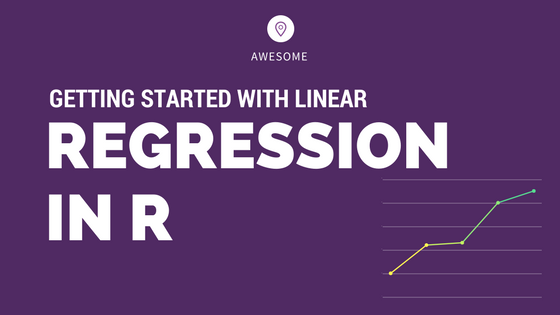## Complete Introduction to Linear Regression in R

Linear regression is used to predict the value of a continuous variable Y based on one or more input predictor variables X. The aim is to establish a mathematical formula between the the response variable (Y) and the predictor variables (Xs). You can use this formula to predict Y, when only X values are known. …